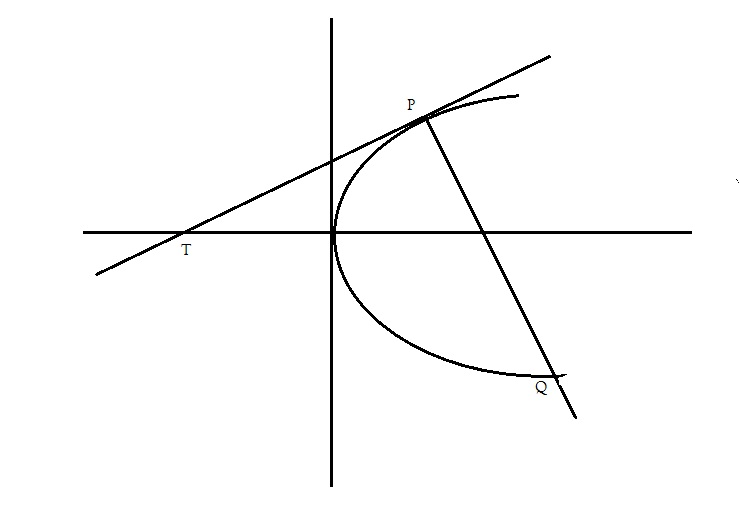#### Thank you for registering.

One of our academic counsellors will contact you within 1 working day.

Please check your email for login details.

Click to Chat

1800-1023-196

+91 7353221155

CART 0

• 0
MY CART (5)

Use Coupon: CART20 and get 20% off on all online Study Material

ITEM
DETAILS
MRP
DISCOUNT
FINAL PRICE
Total Price: Rs.

There are no items in this cart.
Continue Shopping

# in the parabola y^2 =4ax ,the tangent at the point P whose abcissa is equal to the latus rectum meets the axis in T and the normal at P cuts the parabola again in Q.prove PT:PQ=4:5

## 2 Answers

9 years ago

if abcissa of p is equal to latus rectum i.e 4a

then it's y coordinate will be y^2=4a(4a)       y=4a

hence pt p is(4a,4a)

now tangent at any pt (x1,y1) for parabola is

y.y1=2a(x+x1)

y(4a)=2a(x+4a)

it meet the axis at y=0 and x=-4a    T(-4a,0)

distance PT=((4a+4a)^2+(4a-0)^2)^1/2=4a(5)^1/2      ............(1)

now normal at any pt(x1,y1)is

y-y1=-y1/2a(x-x1)

y-4a=-2(x-4a)        2x+y=12a

solving this eq. with y^2=4ax we get 2 pts.(4a,4a) and(9a,-6a)     Q(9a,-6a)

distance PQ=((4a-9a)^2+(4a+6a)^2)^1/2=5a(5)^1/2      .................(2)

(1)/(2)=4/5    hence proved

if u understand the sol. please click on like it9 years ago

Hi Rohit,

Refer the diagram below:Here let P be (at12,2at1) and let Q be (at22,2at2)

Equtation of tangent at P is t1*y = x + at12. So point T is (-at12,0) [obtained by putting y=0 in the tangent equation]

Also the slope of the line PQ is 2/(t1+t2) --------------{Using y2-y1/x2-x1}.

As PQ is the mormal at P, we have slope of normal at P is -t1-------(As slope of tangent is 1/t1 from tangent equation)

So 2/(t1+t2) = -t1.

This will give t2 = -t1 - 2/t1.

Hence

t2 + t1 = -2/t1 --------------------(A) and

t2 - t1 = -2*t1 - 2/t1 -------------(B)

Now use distance formula for PT, and PQ and take the ratio, you will get an expression interms of t1 and t2.

Use the relation in (A) and (B) and substitute, you will get

PT/PQ = t12/2(t1+1/t1) -------------- (1)

As the abscissa of P is equal to the latus rectum = 4a, we get the value of t1 = 2.

Substitute that in (1), and you have PT/PQ = 2/(2+1/2) = 4/5.

Hope that helps.

All the Best,

Regards,

Ashwin (IIT Madras).

## ASK QUESTION

Get your questions answered by the expert for free